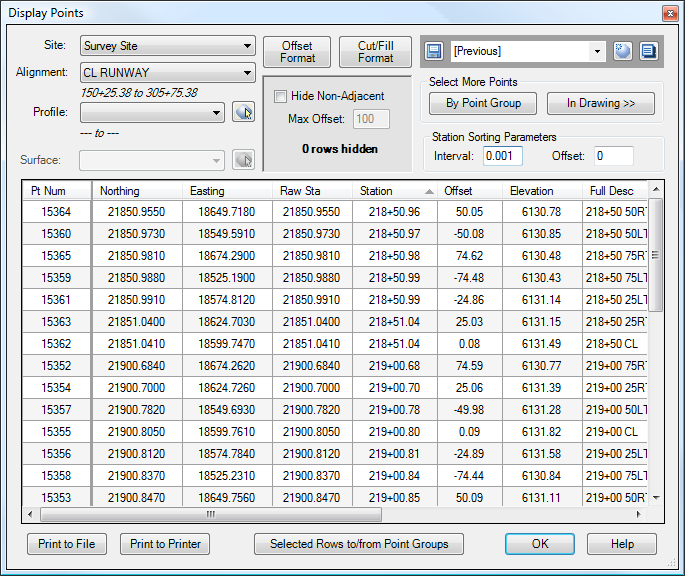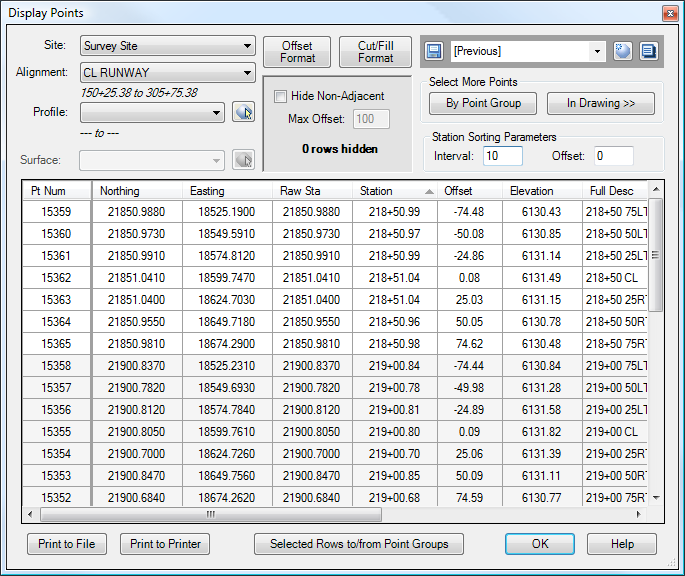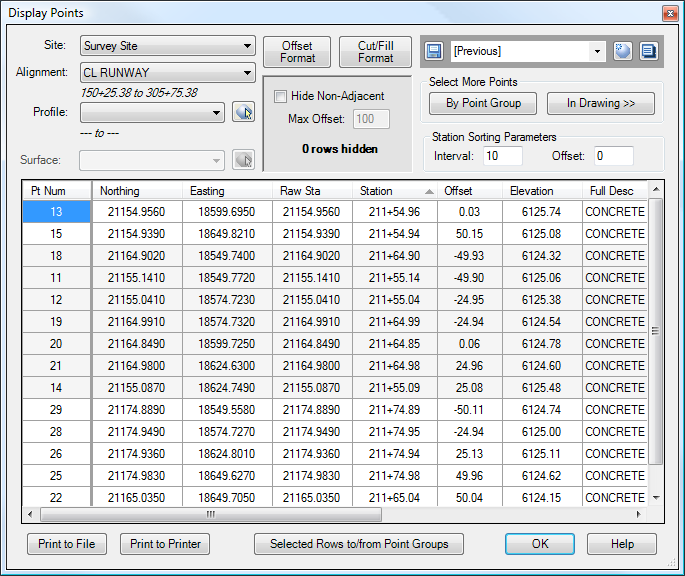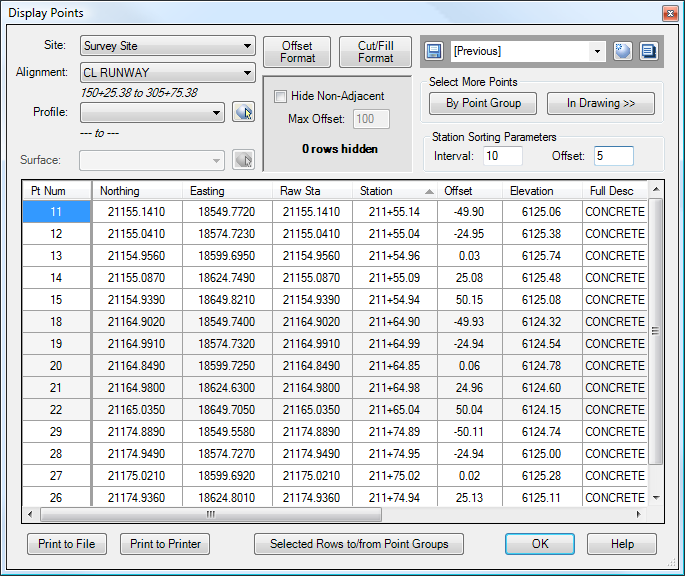Station Sorting Parameters

Description

The Station Sorting Parameters may be used when sorting Cogo Points by Station or Raw Station, and provide a way to control the way points are listed in the grid view.

In general, when sorting by Station, two points at the same station are sorted by offset. However, sometimes, we may have points that are not exactly on even stations, for example, when we have a bunch of points that a surveyor has shot in the field. Since some shots were taken slightly before the even station, and some points after, the points can end up in an undesirable order. That can be seen in the image below. Note how the offsets do not proceed in any consistent order.However, by specifying a Sorting Interval, the station for each point will be rounded to the nearest interval, and this rounded value will be used for sorting. The grid below shows the same data, but with a Station Sorting Interval of 10 feet. Note how the points at each station now proceed in a smooth order from far left to far right at each station.Sometimes, however, simply specifying an Interval may not work. For example, consider the image below. The Interval is set to 10 feet, but the points are being mixed-up. Since each station is being rounded to the nearest 10-foot interval, Point 11 (at Sta. 211+55.14) is being grouped together with Point 18 (at Sta. 211+64.90).The Offset provides a way to deal with this problem. This Offset is added to the station value before it is rounded. In the diagram below, the Offset is set to 5 feet, which fixes the problem.DisplayPoints